# Selina Concise Mathematics Class 9 ICSE Solutions Trigonometrical Ratios of Standard Angles

## Selina Concise Mathematics Class 9 ICSE Solutions Trigonometrical Ratios of Standard Angles [Including Evaluation of an Expression Involving Trigonometric Ratios]

APlusTopper.com provides step by step solutions for Selina Concise Mathematics Class 9 ICSE Solutions Chapter 23 Trigonometrical Ratios of Standard Angles [Including Evaluation of an Expression Involving Trigonometric Ratios]. You can download the Selina Concise Mathematics ICSE Solutions for Class 9 with Free PDF download option. Selina Publishers Concise Mathematics for Class 9 ICSE Solutions all questions are solved and explained by expert mathematic teachers as per ICSE board guidelines.

Selina ICSE Solutions for Class 9 Maths Chapter 23 Trigonometrical Ratios of Standard Angles [Including Evaluation of an Expression Involving Trigonometric Ratios]

Exercise 23(A)

Solution 1: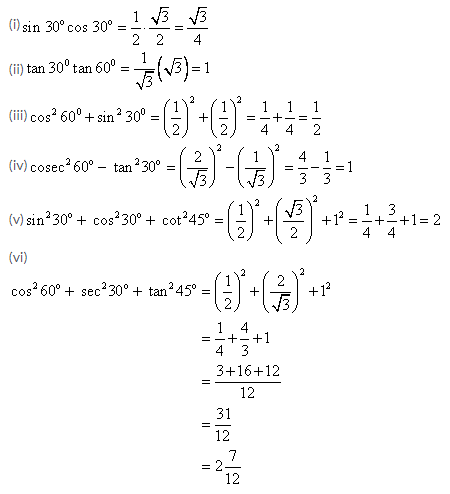Solution 2:Solution 3: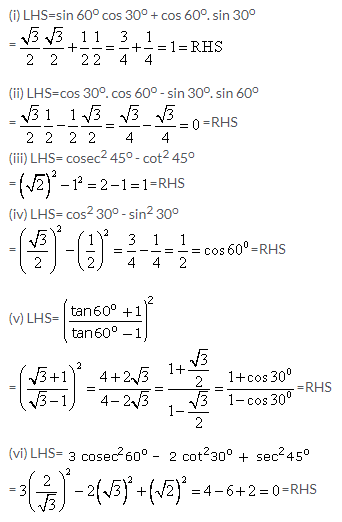Solution 4: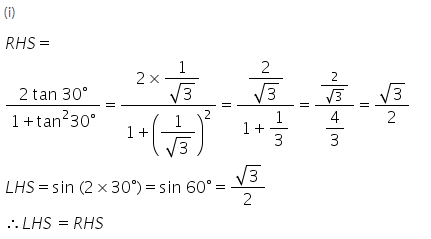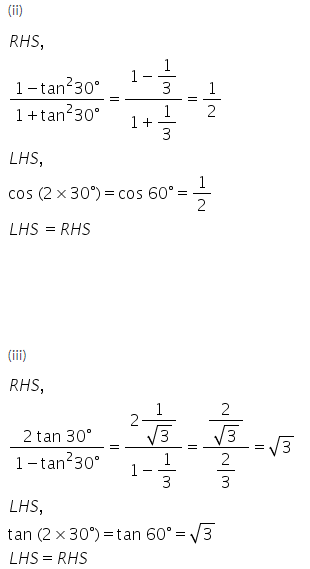Solution 5:Solution 6: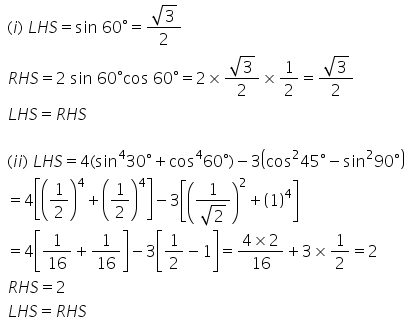Solution 7: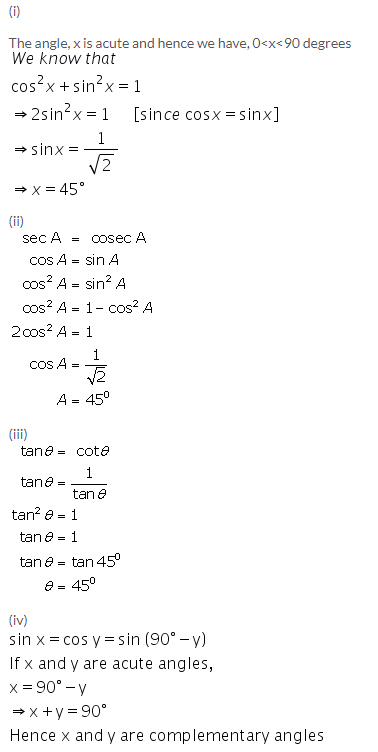Solution 8: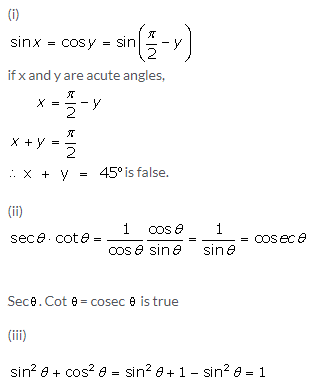Solution 9:
(i) For acute angles, remember what sine means: opposite over hypotenuse. If we increase the angle, then the opposite side gets larger. That means “opposite/hypotenuse” gets larger or increases.
(ii) For acute angles, remember what cosine means: base over hypotenuse. If we increase the angle, then the hypotenuse side gets larger. That means “base/hypotenuse” gets smaller or decreases.
(iii) For acute angles, remember what tangent means: opposite over base. If we decrease the angle, then the opposite side gets smaller. That means “opposite /base” gets decreases.

Solution 10: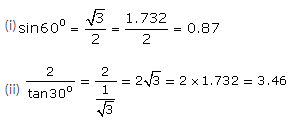Solution 11: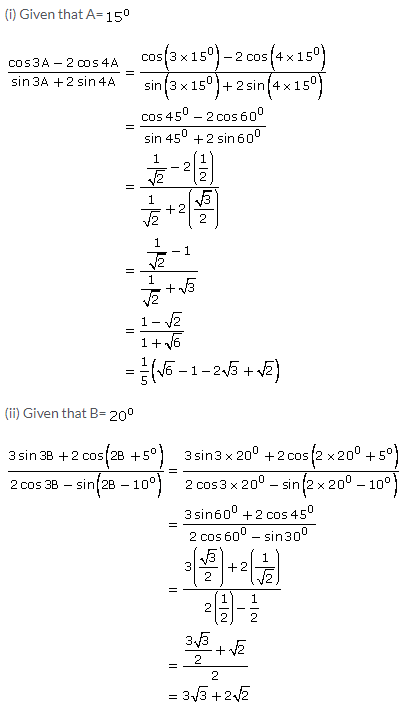Exercise 23(B)

Solution 1: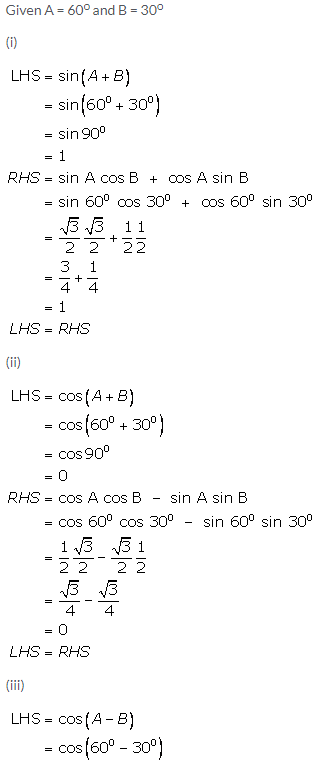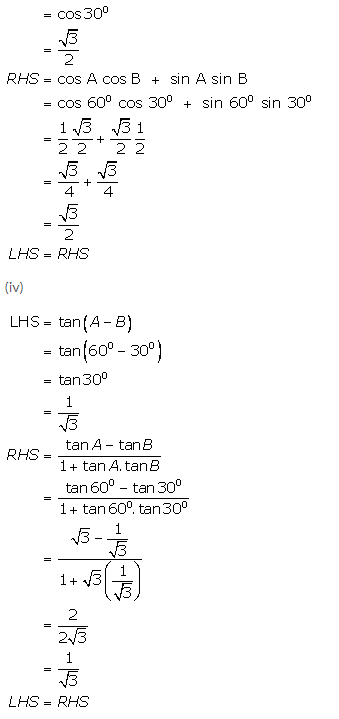Solution 2: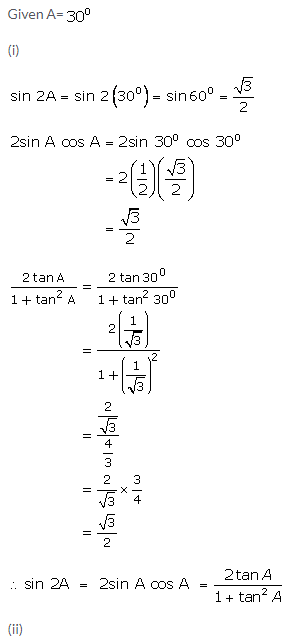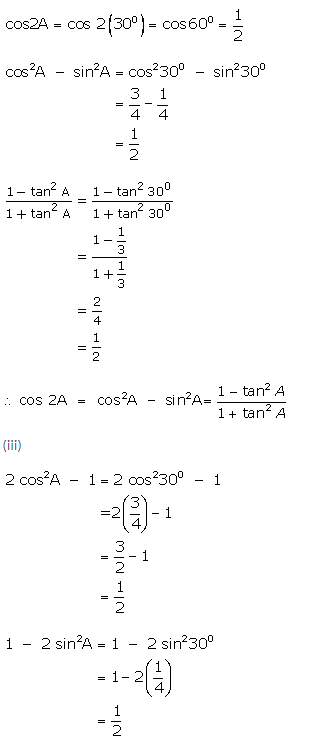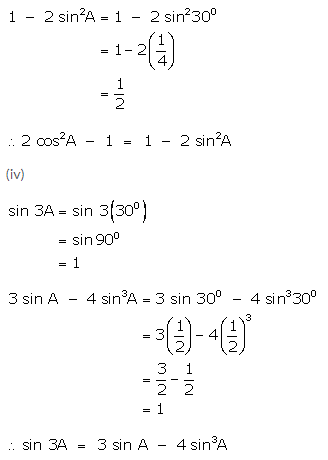Solution 3:Solution 4: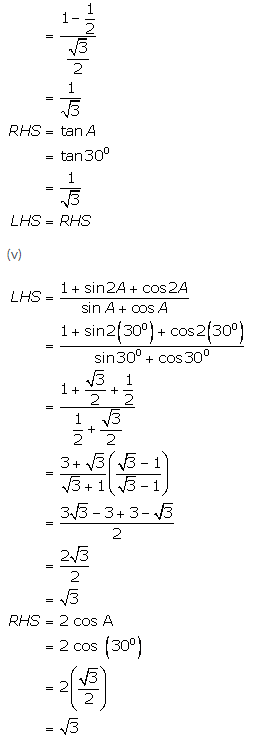Exercise 23(C)

Solution 1: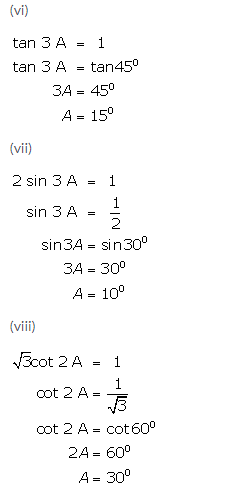Solution 2: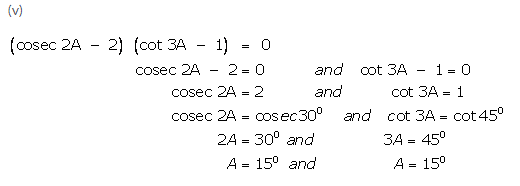Solution 3:Solution 4:Solution 5: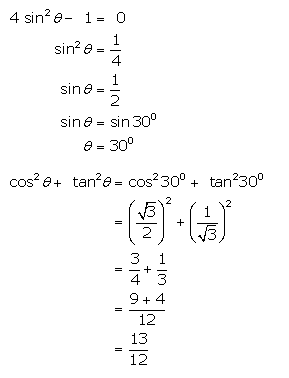Solution 6: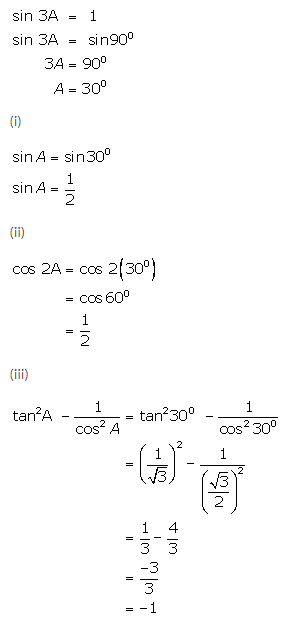Solution 7:Solution 8:Solution 9:Solution 10:Solution 11: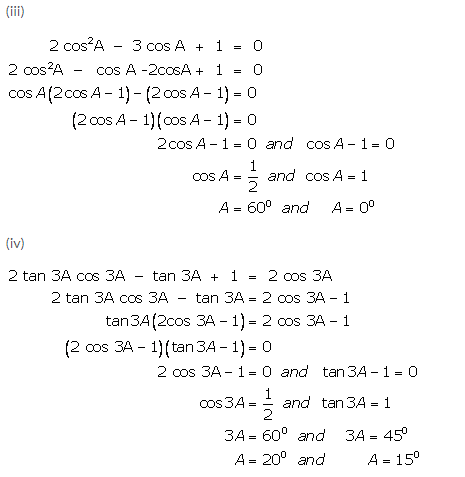Solution 12: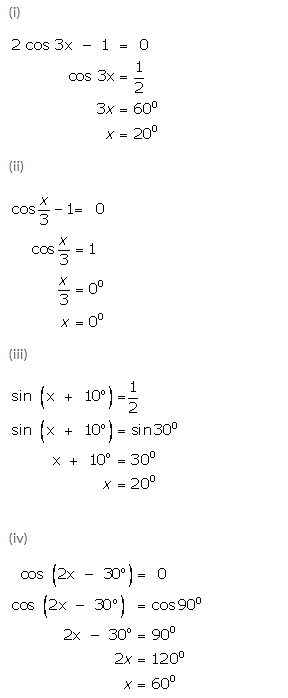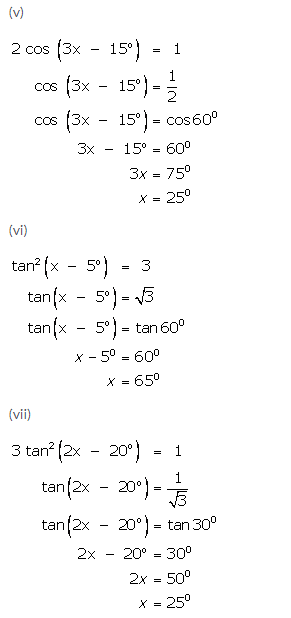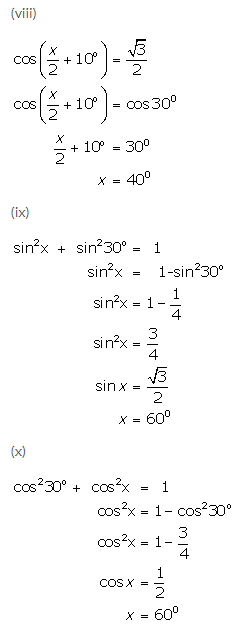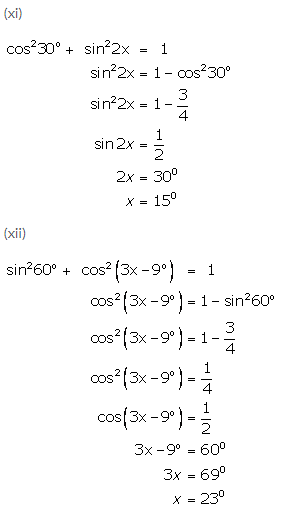Solution 13:Solution 14:Solution 15: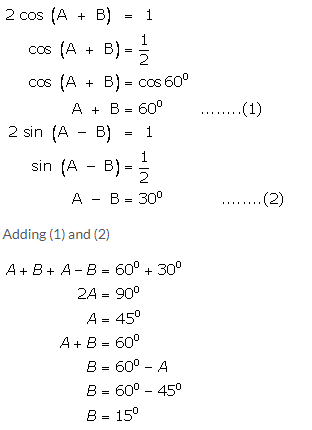More Resources for Selina Concise Class 9 ICSE Solutions